﻿ Dynamic Analysis of the Shaping Machine Mechanism

### Dynamic Analysis of the Shaping Machine Mechanism

Darina Hroncová, Ingrid Delyová, Peter Frankovský, Anna PuzderováOPEN ACCESSPEER-REVIEWED

## Dynamic Analysis of the Shaping Machine Mechanism

Darina Hroncová1,, Ingrid Delyová1, Peter Frankovský1, Anna Puzderová1

1Department of applied mechanics and mechatronics, Technical University of Košice / Faculty of mechanical engineering, Košice, Slovakia

### Abstract

This paper considers the problem of dynamic analysis of a shaping machine mechanism. Mathematical model for the mechanism was compiled and computer simulation was done in the program MSC Adams/View. The results of the computer simulation are kinematical parameters of individual members of the mechanism.

### At a glance: Figures

123456
Prev Next

• Hroncová, Darina, et al. "Dynamic Analysis of the Shaping Machine Mechanism." American Journal of Mechanical Engineering 1.7 (2013): 370-373.
• Hroncová, D. , Delyová, I. , Frankovský, P. , & Puzderová, A. (2013). Dynamic Analysis of the Shaping Machine Mechanism. American Journal of Mechanical Engineering, 1(7), 370-373.
• Hroncová, Darina, Ingrid Delyová, Peter Frankovský, and Anna Puzderová. "Dynamic Analysis of the Shaping Machine Mechanism." American Journal of Mechanical Engineering 1, no. 7 (2013): 370-373.

 Import into BibTeX Import into EndNote Import into RefMan Import into RefWorks

### 1. Introduction

Development of mechanization and automation of production processes requires the construction of new machines and mechanisms. Today's time forces us to technological development still improve and expedite the creation of new mechanical systems. Computer simulations bring ease to and accelerate the innovation of mechanical systems.

One of the most widely used software for dynamic analysis is MSC ADAMS (Automatic Dynamic Analysis of Mechanical Systems). MSC Adams includes a number of modules. MSC Adams/View module is used to for simulation and visualization of the model and it facilitate the evaluation of the analytical results. With Adams/Solver it forms a tool that allows us to deal with all types of MBS (multi-body systems) .

With the development of society also arises the need in progress in automation and mechanization of production processes. This creates the need for constructing new machinery and equipment. Instruments and machines consist of different mechanisms having substantially different functions and structure. Despite this fact a number of mechanisms have common basic functions and structure.

### 2. Mathematical Model of the Mechanism

The basic and also most universal method for the dynamic analysis of mechanical systems is the method which uses the second ’s law. The mathematical model of a four member mechanism in Figure 1 was derived by using this method. The driving torque Mh is exerted on the driving member 2. The resistive force F acts on the driven member 4 with the known geometric and mass properties. In the dynamical analysis the friction between respective members was neglected [1, 2, 3].

To compile equations of motion we analyze the individual members of the mechanism. The links are represented by forces acting on them.

Member 4 as shown in Figure 2 performs translational movement in the horizontal direction. The equations of motion are in form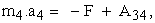(1)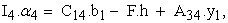(2)

where m4 is the weight, a4 the acceleration, I4 the moment of inertia and α4 the angular acceleration of the member 4.

For member 4 stays that a4y = 0 and α4 = 0. Thus the equations of motion have the form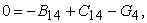(3)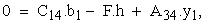(4)

while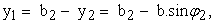(5)

Member 3 describe in Figure 3 of the mechanism performs curvilinear translational movement considering it as a particle. The equations of motion the member 3 are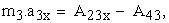(6)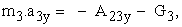(7)

where m3 is the mass of the member 3 and a3x, a3y are components of the acceleration of member 3

By application of the principle of action and reaction stands A43 = A34.

The member 2 performs rotational motion around a fixed axis of rotation perpendicular to the plane of movement and going through point O21. The sliding action of forces acting on member 2 is zero, therefore the equations of motion are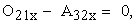(8)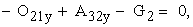(9)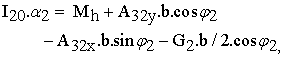(10)

where A32x = A23x, A32y = A23y, I20 is the moment of inertia of member 2, α2 is the angular acceleration of member 2 and m2 is mass of the member 2, while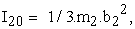(11)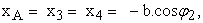(12)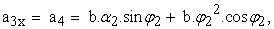(13)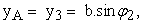(14)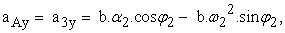(15)

The movement of the system is described by a system of equations. Given the complexity of solving this system of equations the simulation in MS Adams is chosen as the further step in the analysis [8, 9].

### 3. Simulation in MSC Adams/View

For dynamic analysis of individual members of a four-member mechanism we compiled its model in MSC Adams in Figure 5. After running the simulation we can measure the movements of individual members exerted by the driving force and observe the time diagrams of respective kinematical variables [5, 6].

Between members 1 and 2 is rotational joint as well as between members 2 and 3. Member 3 also performs translational movement relative to member 4 therefore these members have also a translational joint. Measures are used to observe the forces in the joint A and 021 and are placed in the respective locations .

The next step is entering the boundary conditions and running the simulation in MSC Adams/View. The result of the simulation is the kinematical parameters of individual members of the mechanism. The individual measures in joints give the respective forces in a defined time interval. In time t = 0,25s the force A = 10,02N in Figure 7 and in Figure 8. The translational joint between member 3 and 4 was measured force A34 = A43 = 9,97N in Figure 9.

Movement of member 3 is given by the displacement y3, velocity v3 and acceleration a3. Their time diagrams are in Figure 10.

Member 2 performs rotational movement around the pivot and is rotated by angle φ21 relative to the base i.e. the member 1. In the Figure 11 there is the time diagram of the rotation angle φ21. The time diagram of the angular velocity ω21 of member 2 is in Figure 12.

Movement of member 4 is given by displacement x4, velocity v4 and acceleration a4.

The time diagram of these parameters is in Figure 13, Figure 14, Figure 15 and Figure 16.

### 4. Conclusion

Program MSC Adams/View makes it easy to analyze complex mechanical systems with multiple degrees of freedom. In the paper one module of the number of modules namely MSC Adams/View was used as a tool which allows a better simulation and visualization of the model and easier evaluation of the results obtained. With the module Adams/Solver they represent a tool that is able to addresses various types of mechanisms with many degrees of freedom. The results obtained by the simulation of four-member mechanism of the shaping machine were processed by the postprocessor program of MSC Adams/View.

### Acknowledgement

This work was supported by grant projects VEGA No. 1/0289/11, VEGA No. 1/1205/12 and grant projects VEGA No. 1/0937/12 and APVV-0091-11.

### References

  Drahoš, P., Kutiš, V., Dúbravský, J., Sedlár, T., 2011. Design and Simulation of SMA Actuator, International Review of Automatic Control. Vol. 4, No. 4 (2011), p. 588-593.In article  Hanzel, J., Jurišica, L., Kľúčik, M., Duchoň, F., 2011. Identification of the Experimental Ultrasonic System Parameters, in”AD ALTA” Journal of Interdisciplinary Research. Vol. 1, Iss. 2 (2011), p. 138-141.In article  Hanzel, J., Kľúčik, M., Jurišica, L., Vitko, A., 2011. Identification Based Model of Ultrasonic Sensor, in “Research and Education in Robotics - EUROBOT 2011”. Prague, Czech Republic, Heidelberg, Springer, pp. 144-157.In article CrossRef  Novotný, L., Tsunori, M., 2011. Creation of Imperfections for Welding Simulations, in “CMES: Computer Modeling in Engineering & Sciences,” Vol.82, Nos.3&4, p. 253-264.In article  Pásztó, P., Hubinský, P., 2010. “Application of a Visual System for a Mobile Robot Navigation (OpenCV),” in Process Control 9th International Conference. University of Pardubice, Kouty nad Desnou, Česko, paper C015a.In article  Delyová, I., Frankovský, P., Hroncová, D., 2011. Kinematic analysis of movement of a point of a simple mechanism,” 4th International Conference Modelling of mechanical and mechatronics systems, KAMaM, TU Košice, Herľany, Slovakia.In article  Segľa, Š., Segľa, J., 2011. Modelling and Optimization of Vehicle suspension with Magnetorheological Dampers, 7th International Conference Dynamics of Rigid and Deformable Bodies 2008, FVTM UJEP Ústí nad Labem , Česko.In article  Skočilas, J., Skočilasová, B., Soukup, J., 2011. Investigation and vibration of vehicle mechanical model. In: Dynamika tuhých a deformovatelných těles 2011 - Sborník přednášek z 9. mezinárodní konference v Ústí nad Labem, FVTM UJEP, Ústí nad Labem, Česko.In article  Svoboda, M., Soukup, J., 2011. „Analytical solutions of motion equations of railway car, determination of bazic geometric, mass and stiffness parameters,“ Dynamika tuhých a deformovatelných těles 2011 - Sborník přednášek z 9. mezinárodní konference. FVTM UJEP, Ústí nad Labem, Česko.In article